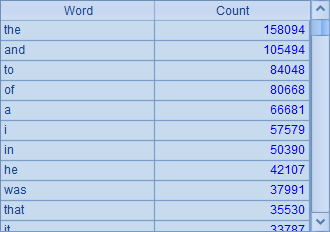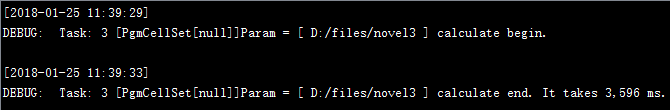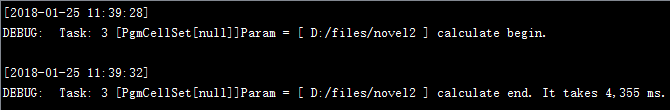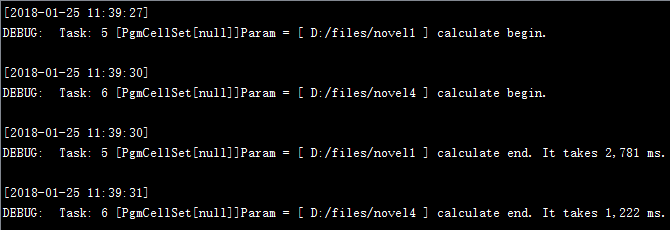# 趣味集算：wordcount## 先从单线程开始。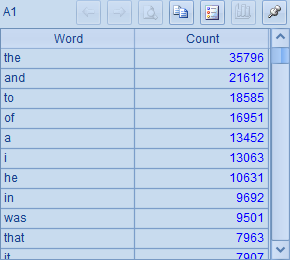A B C 1 =directory@p("D:/files/novel") [] =now() 2 for A1 =file(A2).read().words() 3 =B2.groups(lower(~):Word;count(~):Count) 4 >B1=B1|[B3] 5 =B1.merge(Word) =A5.groups@o(Word;sum(Count):Count).sort@z(Count) =interval@ms(C1,now())B3中统计出当前文档中每个单词出现的次数，统计时将单词转换为小写字母，以避免大小写变化的影响。结果将按照单词的字典顺序排列：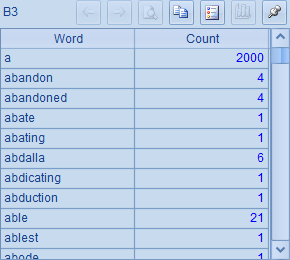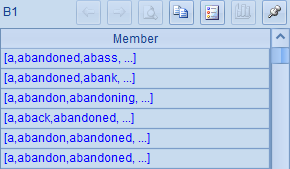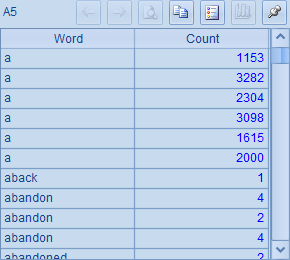B5中，将归并后的统计结果按每个单词聚合计算，再将结果按Count降序排列，可以得到和前面单条语句时相同的结果：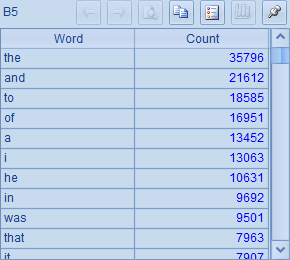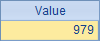## 搞完单线程，我们再来试试多线程的搞法。

 A B C 1 =directory@p("D:/files/novel") =now() 2 fork A1 =file(A2).read().words() 3 =B2.groups(lower(~):Word;count(~):Count) 4 =A2.merge(Word) =A4.groups@o(Word;sum(Count):Count).sort@z(Count) =interval@ms(C1,now())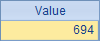## 搞完多线程，现来搞集群。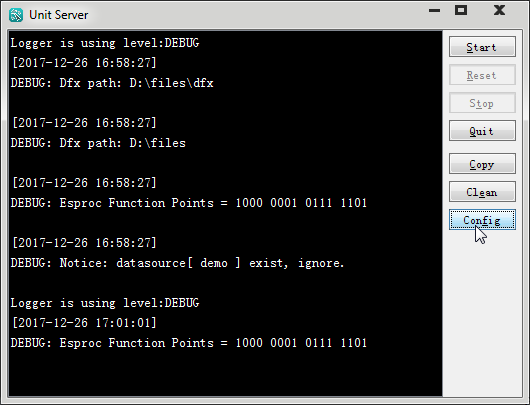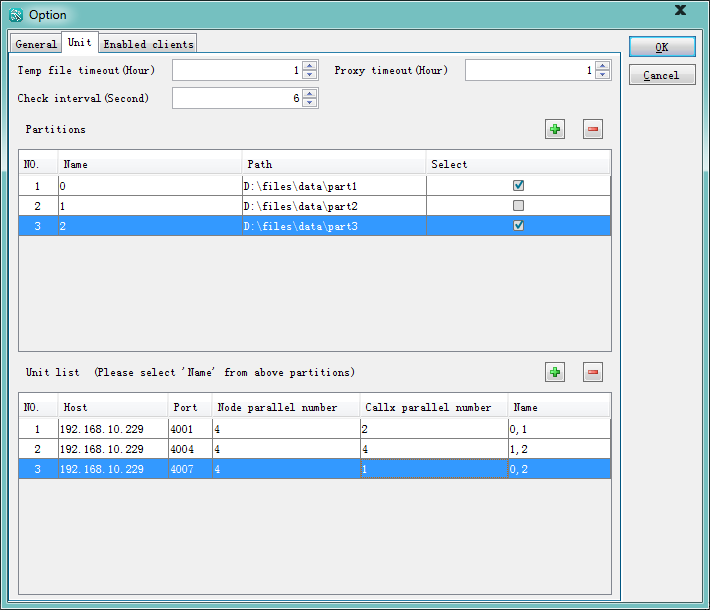A B C 1 [192.168.10.229:4001,192.168.10.229:4004,192.168.10.229:4007] [D:/files/novel1,D:/files/novel2, D:/files/novel3,D:/files/novel4] 2 fork B1;A1 =directory@p(A2) 3 fork B2 =file(B3).read().words() 4 =C3.groups(lower(~):Word;count(~):Count) 5 return B3.merge(Word) 6 =A2.merge(Word) =A6.groups@o(Word;sum(Count):Count).sort@z(Count)